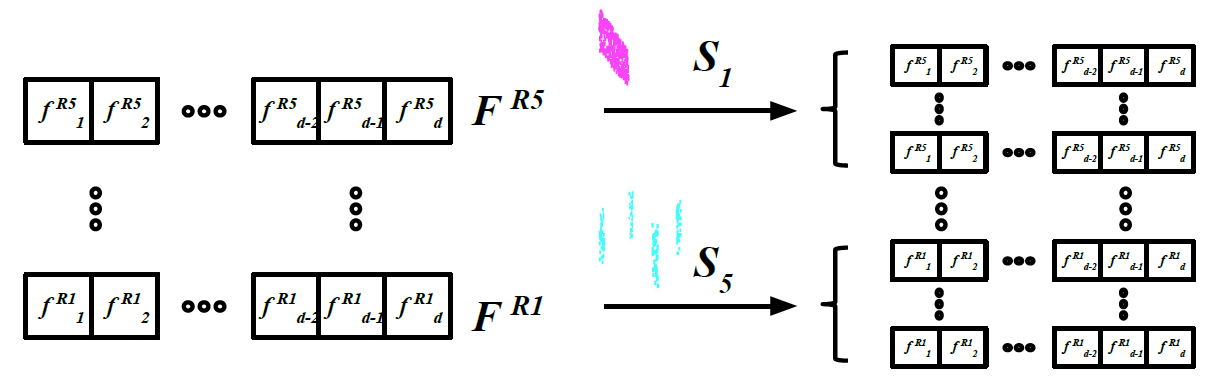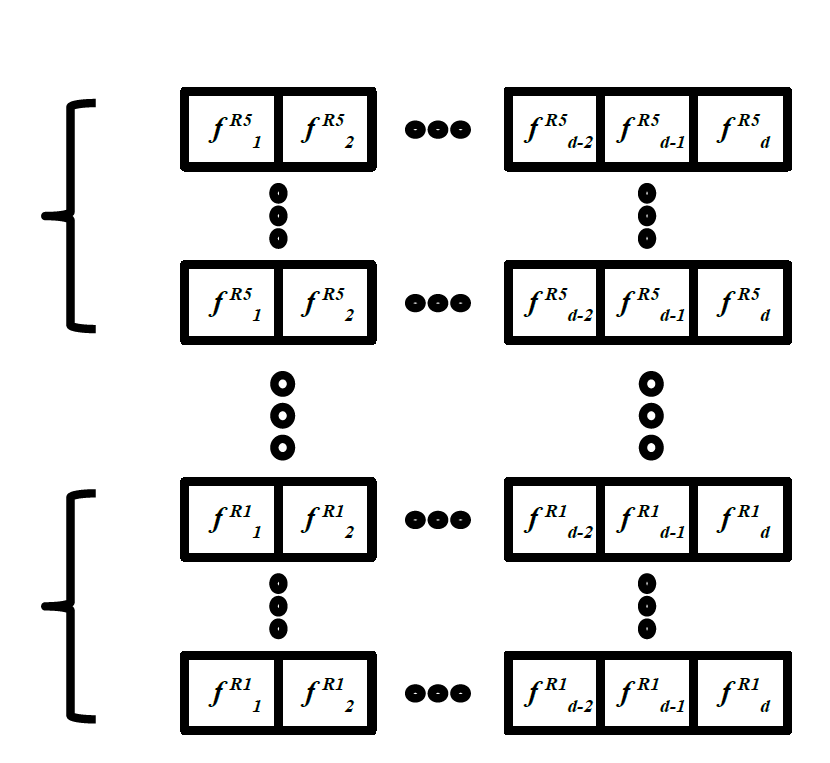2017-06-07 23:56:45 heyc861221 阅读数 1642
• ###### 深度学习框架Tensorflow案例实战视频课程

深度学习框架Tensorflow案例实战视频培训课程概况： Tensorflow是谷歌开源的深度学习（包括机器学习）框架，伴随着人工智能业的兴盛其大名早已响彻云霄。本课程从Tensorflow安装开始讲起，从基本计算结构到深度学习各大神经网络，全程案例代码实战，一步步带大家入门如何使用深度学习框架Tensorflow，玩转Tensorflow模型训练、等所有知识点。

30268 人正在学习 去看看 唐宇迪

### 云深度学习平台设计

• 屏蔽硬件资源保证开箱即用
• 缩短业务环境部署和启动时间
• 提供“无限”的存储和计算能力
• 实现多租户隔离保证数据安全
• 实现错误容忍和自动故障迁移
• 提高集群利用率和降低性能损耗

• 支持通用GPU等异构化硬件
• 支持主流的深度学习框架接口
• 支持无人值守的超参数自动调优
• 支持从模型训练到上线的工作流

### 云深度学习平台架构### 云深度学习平台实现### 总结2017中国人工智能大会（CCAI 2017）| 7月22日-23日 杭州

- 40位以上实力讲师
- 8场权威专家主题报告
- 4场开放式专题研讨会
- 超过100家媒体报道
- 超过2000位技术精英和专业人士参会2018-09-14 10:58:20 oliongs 阅读数 8056
• ###### 深度学习框架Tensorflow案例实战视频课程

深度学习框架Tensorflow案例实战视频培训课程概况： Tensorflow是谷歌开源的深度学习（包括机器学习）框架，伴随着人工智能业的兴盛其大名早已响彻云霄。本课程从Tensorflow安装开始讲起，从基本计算结构到深度学习各大神经网络，全程案例代码实战，一步步带大家入门如何使用深度学习框架Tensorflow，玩转Tensorflow模型训练、等所有知识点。

30268 人正在学习 去看看 唐宇迪

# PointNet

（PointNet: Deep Learning on Point Sets for 3D Classification and Segmentation）

（作为第一个直接处理点云的深度学习框架，相关介绍太多了，不再赘述）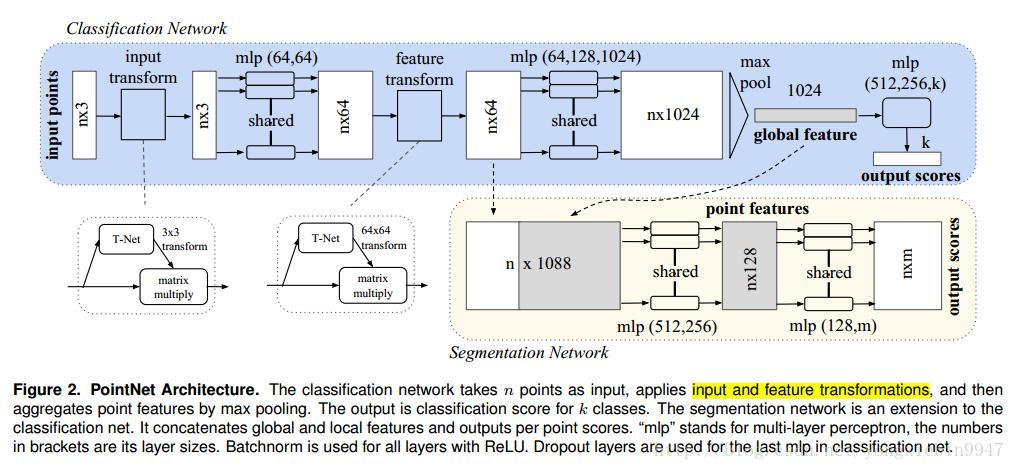# PointNet++

（PointNet++： Deep Hierarchical Feature Learning on Point Sets in a Metric Space）

## 1.文章主要解决两个问题

• ### 怎么组合点云集的局部特征

local region中的坐标首先被转换到相对于中点的坐标系中，使用相对坐标系，我们可以捕获到局部区域中点到点的关系。

Multi-scale grouping (MSG)：每个尺度使用PointNet，然后将多尺度特征融合到一起。但是计算量比较大。

Multi-resolution grouping (MRG)：MRG特征由两个向量组成，左边的使用区域抽象层汇总每个子区域的特征，右边的使用PointNet直接处理所有原始点数据。当局部区域密度低时，左边的特征不如右边的可靠，因为它包含更加稀疏的点，并且更多收到了采样的影响。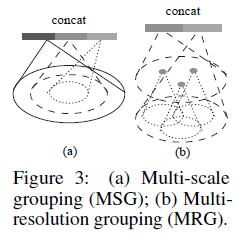## 2.整体框架如下：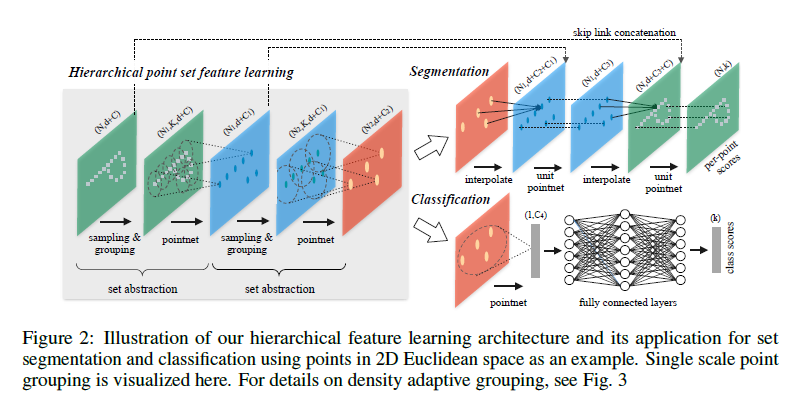set abstraction: 主要分为上面提到的Sampling layer、Grouping layer 和PointNet layer

# 3.总结：

https://blog.csdn.net/sunfei05/article/details/80451808

https://blog.csdn.net/qq_15332903/article/details/80261951

https://www.cnblogs.com/lainey/p/8625141.html

2018-10-10 16:28:17 qq_28863845 阅读数 3041
• ###### 深度学习框架Tensorflow案例实战视频课程

深度学习框架Tensorflow案例实战视频培训课程概况： Tensorflow是谷歌开源的深度学习（包括机器学习）框架，伴随着人工智能业的兴盛其大名早已响彻云霄。本课程从Tensorflow安装开始讲起，从基本计算结构到深度学习各大神经网络，全程案例代码实战，一步步带大家入门如何使用深度学习框架Tensorflow，玩转Tensorflow模型训练、等所有知识点。

30268 人正在学习 去看看 唐宇迪

## 使用华为云深度学习服务完成kaggle猫狗识别竞赛

1. kaggle猫狗竞赛kernel第一名的代码
2. Tensorflow官网代码
3. 华为云DLS服务github代码

### 1. 环境配置与数据集处理

• 首先我们需要从kaggle上面找到猫狗竞赛的页面，下载数据集压缩文件all.zip，大概853MB，解压两次后可以得到两个文件夹test和train，以及一个sample_submission.csv文件，test里面有12500张没有标签的测试图片，train中有带标签的25000张图片，猫狗各12500张，且按照顺序排好了。
• 在桶的目录下创建code、log、model、train、test五个文件夹。
• 使用华为云OBS客户端上传之前解压的图片，我是将解压后的图片上传的，所以需要一定的时间，文件位置为桶的目录下创建的train和test文件夹。
• 创建开发环境，添加jupyter notebook

### 2. 代码-库的导入

``````from __future__ import division, absolute_import, print_function
import os, cv2, random
import pandas as pd
import tensorflow as tf
import numpy as np
import moxing as mox
from tensorflow import keras
from tensorflow.python.keras import layers
``````

### 3. 代码-定义常量以及运行时参数

``````# 使用tf.app.flags定义参数，运行时可以指定
tf.app.flags.DEFINE_string('data_url', 's3://dls-dogcat/input/', 'Dataset')
tf.app.flags.DEFINE_string('train_url', 's3://dls-dogcat/model/', 'Model_dir')
tf.app.flags.DEFINE_string('log_url', 's3://dls-dogcat/log/', 'Log_dir')

flags = tf.app.flags.FLAGS
TRAIN_DIR = os.path.join(flags.data_url, 'train/')
TEST_DIR = os.path.join(flags.data_url, 'test/')
MODEL_DIR = flags.train_url
LOG_DIR = flags.log_url

# 注释掉的部分用于测试
# TRAIN_DIR = 's3://dls-dogcat/input/train/'
# TEST_DIR = 's3://dls-dogcat/input/test/'
# MODEL_DIR = 's3://dls-dogcat/model/'
# LOG_DIR = 's3://dls-dogcat/log/'

# 这里获取文件夹下所有文件名称使用mox的函数，或者使用tf.gfile，不能使用os，测试数据需要按照名称顺序排列，
# 我在ubuntu下测试时发现文件名的排列规则是按照字符顺序，所以这里使用循环可以避免这个问题
train_image_url = [TRAIN_DIR + i for i in mox.file.list_directory(TRAIN_DIR)]
test_image_url = []
for i in range(1, 12501):
path = TEST_DIR + str(i) + '.jpg'
test_image_url.append(path)
print('Train images : {}, Test images : {}'.format(len(train_image_url), len(test_image_url)))

# 图片压缩为64*64大小，彩色图片3或灰度图片1
IMAGE_SIZE = 64
CHANNELS = 3

# batch大小16，epoch为30，但是一般不会跑完30轮，因为后面我设置了earlystop
BATCH_SIZE = 16
EPOCH = 30

# STEPS_PER_EPOCH后面实际没有使用，打乱训练集顺序
STEPS_PER_EPOCH = len(train_image_url) // BATCH_SIZE
random.shuffle(train_image_url)

# 使用mox获取文件夹下所有文件名
train_images = [TRAIN_DIR + i for i in mox.file.list_directory(TRAIN_DIR)]
test_images = [TEST_DIR + i for i in mox.file.list_directory(TEST_DIR)]

STEPS_PER_EPOCH = len(train_images) // BATCH_SIZE

# 数据集打乱
random.shuffle(train_images)

# 定义读取图片的函数，并且resize大小，设置为灰度图片或彩色，与上面CHANNELS对应即可
return cv2.resize(img, (IMAGE_SIZE, IMAGE_SIZE), interpolation=cv2.INTER_CUBIC)

# 将图片数据存入array中，同时除以255进行归一化
def prep_data(images):
count = len(images)
data = np.ndarray((count, IMAGE_SIZE, IMAGE_SIZE, CHANNELS), dtype=np.float32)
for i, image_file in enumerate(images):
data[i] = image / 255.0
if i%1000 == 0: print('Processed {} of {}'.format(i, count))
return data

train_set = prep_data(train_image_url)
test_set = prep_data(test_image_url)
print('Train_set shape: {}, Test_set shape: {}'.format(train_set.shape, test_set.shape))

# 获取训练集对应的label，就是将文件路径切分，找出是否包含dog或者cat，若是dog则写入1，否则0
train_labels = np.ndarray((len(train_image_url), 1), np.int32)
for i, url in enumerate(train_image_url):
tag = url.split('.')[-3].split('/')[-1]
if tag == 'dog':
train_labels[i] = 1
elif tag == 'cat':
train_labels[i] = 0

print('Train_labels shape: {}'.format(train_labels.shape))
``````

### 4. 代码-CNN构建

``````# 添加一些参数，便于调整神经网络，包括units，ksize，strides
f1, f2, f3, f4 = 32, 64, 128, 256
k1, k2, k3, k4 = 5, 5, 5, 5
s1, s2, s3, s4 = 2, 2, 2, 2
c1, c2, c3, c4 = 2, 2, 2, 2
fc1, fc2, fc3, fc4 = 512, 256, 128, 128

# 有三层（卷积层+最大池化+Dropout），然后Flatten层，再是全连接层，再是输出层
# 前面三层使用relu激活，drop概率0.5，全连接层drop概率0.5，最后输出层使用sigmoid激活
# 损失函数binary_crossentropy，优化器rmsprop
# 可优化部分：
# 1.增大内存，受限于内存，总参数在大概600000以上时，32GB内存会不够用，增加内存可以提升CNN的复杂度，也可以使用128大小的图片；
# 2.使用model_to_estimator将model转为estimator，然后使用GPU训练，可以提升训练速度；
# 3.使用vgg16取代前三层卷积层，可以实现更好的效果。

def cnn_model():
model = keras.Sequential([
layers.Conv2D(f1, k1, c1, padding='SAME', activation=tf.nn.relu, input_shape=(IMAGE_SIZE, IMAGE_SIZE, CHANNELS)),
layers.Dropout(0.5),

layers.Dropout(0.5),

layers.Dropout(0.5),

#         layers.Conv2D(f4, k4, c4, padding='SAME', activation=tf.nn.relu),
#         layers.Dropout(0.5),

layers.Flatten(),
layers.Dense(fc1, activation=tf.nn.relu),
layers.Dropout(0.5),
layers.Dense(fc2, activation=tf.nn.relu),
layers.Dropout(0.5),
#         layers.Dense(fc3, activation=tf.nn.relu),
#         layers.Dropout(0.5),
#         layers.Dense(fc4, activation=tf.nn.relu),
#         layers.Dropout(0.5),

layers.Dense(1, activation=tf.nn.sigmoid)
])
model.compile(loss='binary_crossentropy',
optimizer=tf.keras.optimizers.RMSprop(lr=1e-4),
metrics=['accuracy'])
return model

model = cnn_model()
model.summary()
``````

### 5. 代码-训练模型

``````# 设置PrintLoss callback在训练过程中打印损失和准确率
# 设置early_stop避免过拟合
# 设置tensorboard callback记录训练过程中loss、acc、val_loss、val_acc
# 设置checkpoint_callback保存训练过程中的模型
# 由于cp_callback、tb_callback不能直接写入，所以在华为云上实际运行时没有使用，如果跑在自己的机器上可以使用
early_stop = tf.keras.callbacks.EarlyStopping(monitor='val_loss',
patience=3,
mode='auto')

checkpoint_path = MODEL_DIR + "cp-{epoch:04d}.ckpt"
cp_callback = tf.keras.callbacks.ModelCheckpoint(checkpoint_path,
verbose=1,
save_weights_only=True,
period=5)

tb_callback = tf.keras.callbacks.TensorBoard(log_dir=LOG_DIR, batch_size=BATCH_SIZE)

class PrintLoss(keras.callbacks.Callback):
def on_epoch_end(self, epoch, logs):
print('Epoch: {:03d} - loss: {:.5f} - acc: {:.5f} - val_loss: {:.5f} - val_acc: {:.5f}'.format(epoch + 1,
logs['loss'],
logs['acc'],
logs['val_loss'],
logs['val_acc']))

# 设置validation_split获取验证集损失
history = model.fit(train_set,
train_labels,
epochs=EPOCH,
batch_size=BATCH_SIZE,
validation_split=0.2,
verbose=0,
callbacks=[early_stop, cp_callback, tb_callback, PrintLoss()])

# 随便测试一下模型对训练集前3000图片的准确率
model.evaluate(train_set[:3000], train_labels[:3000])
``````

### 6. 代码-预测与写入

``````# 调用predict进行预测
predictions = model.predict(test_set)

import pandas as pd
# path = os.path.join(flags.log_dir, 'submission.csv')
path = 's3://dls-dogcat/log/submission.csv'
counter = range(1, len(predictions) + 1)
df = pd.DataFrame({'id': counter, 'label': predictions})
# df['label'] = df['label'].map(lambda x: x.lstrip('[').rstrip(']')).astype('float32')
# df.reindex(index=list(range(1, df.shape)))
result = df.to_csv(path_or_buf=None, index=None)
with tf.gfile.Open(path, 'w') as f:
f.write(result)
print('write success')
``````

submission格式有要求，columns为id和label，label列下的数据格式要对，以及向桶中写数据的方式，参考华为云项目在github中的代码

### 7. kaggle分数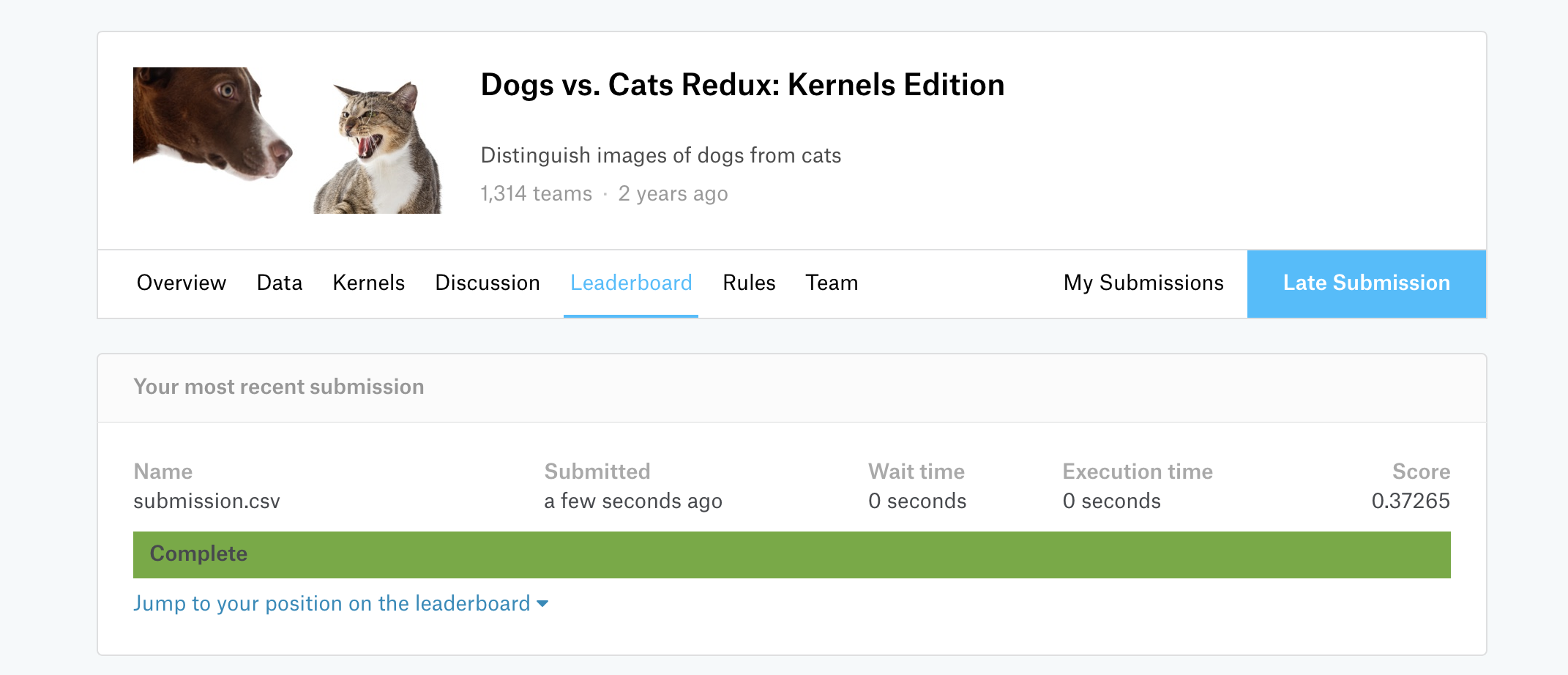## 使用华为云深度学习服务完成kaggle猫狗识别竞赛-进阶版

### 1. 数据处理

``````# 前面的部分代码和keras模式基本相同，将图片数据处理为numpy数组，不同的地方在于这个numpy数组我reshape为二维的了
# coding=utf-8
from __future__ import division, absolute_import, print_function
import os, cv2, random
import pandas as pd
import tensorflow as tf
import numpy as np
import moxing as mox
import matplotlib.pyplot as plt
import moxing.tensorflow as mtf
from sklearn.model_selection import train_test_split
from tensorflow import keras
from tensorflow.python.keras import layers
slim = tf.contrib.slim

TRAIN_DIR = 's3://dls-dogcat/input/train/'
TEST_DIR = 's3://dls-dogcat/input/test/'
OUTPUT_DIR = 's3://dls-dogcat/input/128'
TRAIN64 = 'train64.tfrecord'
EVAL64 = 'eval64.tfrecord'
TEST64 = 'test64.tfrecord'

TRAIN128 = 'train128.tfrecord'
EVAL128 = 'eval128.tfrecord'
TEST128 = 'test128.tfrecord'

train_image_url = [TRAIN_DIR + i for i in mox.file.list_directory(TRAIN_DIR)]

IMAGE_SIZE = 64
CHANNELS = 3

random.shuffle(train_image_url)

TRAIN_SIZE = len(train_image_url)
TEST_SIZE = len(tf.gfile.ListDirectory(TEST_DIR))
test_image_url = []
for i in range(1, TEST_SIZE + 1):
path = TEST_DIR + str(i) + '.jpg'
test_image_url.append(path)
print('Train images : {}, Test images : {}'.format(TRAIN_SIZE, TEST_SIZE))

return cv2.resize(img, (IMAGE_SIZE, IMAGE_SIZE), interpolation=cv2.INTER_CUBIC)

def prep_data(images):
count = len(images)
data = np.ndarray((count, IMAGE_SIZE * IMAGE_SIZE * CHANNELS), dtype=np.float32) # data也要改变对应下面
for i, image_file in enumerate(images):
image = image.reshape(-1) # 为了使用tfrecord的floatlist，我reshape了一下，其实可以使用byteslist，就不用reshape
data[i] = image / 255.0
if i%1000 == 0: print('Processed {} of {}'.format(i, count))
return data

train_set = prep_data(train_image_url)
test_set = prep_data(test_image_url)
print('Train_set shape: {}, Test_set shape: {}'.format(train_set.shape, test_set.shape))

train_labels = np.ndarray((len(train_image_url), 1), np.int64)
for i, url in enumerate(train_image_url):
tag = url.split('.')[-3].split('/')[-1]
if tag == 'dog':
train_labels[i] = 1
elif tag == 'cat':
train_labels[i] = 0
# 在这里划分数据集，80%train， 20%eval
print('Train_labels shape: {}'.format(train_labels.shape))
train_imgs, eval_imgs, train_labels, eval_labels = train_test_split(train_set, train_labels, test_size=0.2)
print(train_imgs.shape, eval_imgs.shape, train_labels.shape, eval_labels.shape)

# 将train和eval的numpy数组写入tfrecord的函数，这里需要定义Features，一个是image保存图片数组，一个是label保存标签（1、0）
# FloatList要求数组为一维的，所以上面我reshape了，如果使用byteslist就不用
def convert_and_encode_to_tfrecord(num_samples, images, labels, output_file):
output_file = os.path.join(OUTPUT_DIR, output_file)
with tf.python_io.TFRecordWriter(output_file) as tfrecord_writer:
for i in range(num_samples):
example = tf.train.Example(features=tf.train.Features(feature={
'image': tf.train.Feature(float_list=tf.train.FloatList(value=images[i])),
'label': tf.train.Feature(int64_list=tf.train.Int64List(value=labels[i]))
}))
tfrecord_writer.write(example.SerializeToString())
# 此处执行完毕会产生train64.tfrecord和eval64.tfrecord两个文件，train0.916GB，eval234MB，tfrecord比源文件大是很正常的
convert_and_encode_to_tfrecord(train_imgs.shape, train_imgs, train_labels, TRAIN64)
convert_and_encode_to_tfrecord(eval_imgs.shape, eval_imgs, eval_labels, EVAL64)

# 同理，这里将test写入tfrecord，但是test没有label，所以只需要一个feature
def convert_test_and_encode_to_tfrecord(num_samples, images, output_file):
output_file = os.path.join(OUTPUT_DIR, output_file)
with tf.python_io.TFRecordWriter(output_file) as tfrecord_writer:
for i in range(num_samples):
example = tf.train.Example(features=tf.train.Features(feature={
'image': tf.train.Feature(float_list=tf.train.FloatList(value=images[i]))
}))
tfrecord_writer.write(example.SerializeToString())
# 大概586MB
convert_test_and_encode_to_tfrecord(test_set.shape, test_set, TEST64)

# 读取tfrecord的数据并显示出来，就可以验证是否正确的存入了数据，使用mox的get_tfrecord，参数照着改就完事了
# 这个方法不能与下面读取test一起使用，因为使用了session
keys_to_features = {
'image': tf.FixedLenFeature((IMAGE_SIZE * IMAGE_SIZE * CHANNELS,), tf.float32, default_value=None),
'label': tf.FixedLenFeature(, tf.int64, default_value=None),
}
# 这里可以实现对一维数组的reshape，我们将数据恢复为彩色图片的三维形式
items_to_handlers = {
'image': slim.tfexample_decoder.Tensor('image', shape=[IMAGE_SIZE, IMAGE_SIZE, CHANNELS]),
'label': slim.tfexample_decoder.Tensor('label', shape=[]),
}
dataset = mtf.get_tfrecord(dataset_dir=dataset_dir,
file_pattern=file_pattern,
num_samples=num_samples,
keys_to_features=keys_to_features,
items_to_handlers=items_to_handlers,
shuffle=False,
num_epochs=1)
image, label = dataset.get(['image', 'label'])
sv = tf.train.Supervisor()
# 这里可以显示图片，20张彩色图片
with sv.managed_session() as sess:
plt.figure()
for i in range(20):
subp = plt.subplot(4, 5, i + 1)
subp.imshow(sess.run(image))
label_eval = sess.run(label)
subp.set_title('label=%s' % (label_eval))
plt.show()

file_pattern='eval*.tfrecord',
num_samples=5000)

keys_to_features = {
'image': tf.FixedLenFeature((IMAGE_SIZE * IMAGE_SIZE * CHANNELS,), tf.float32, default_value=None)
}
items_to_handlers = {
'image': slim.tfexample_decoder.Tensor('image', shape=[IMAGE_SIZE, IMAGE_SIZE, CHANNELS])
}
dataset = mtf.get_tfrecord(dataset_dir=dataset_dir,
file_pattern=file_pattern,
num_samples=num_samples,
keys_to_features=keys_to_features,
items_to_handlers=items_to_handlers,
shuffle=False,
num_epochs=1)
image = dataset.get(['image'])
sv = tf.train.Supervisor()
with sv.managed_session() as sess:
plt.figure()
for i in range(20):
subp = plt.subplot(4, 5, i + 1)
subp.imshow(sess.run(image)) # 注意这个位置，test读出来的形式不一样，需要取image才是正确的图片信息，此处有伏笔
plt.show()

file_pattern='test*.tfrecord',
num_samples=12500)

``````

### 2. 代码

``````# 前面部分不必多说，是一些固定的库以及参数
from __future__ import absolute_import, division, print_function
import os
os.environ.pop('http_proxy', None)
import math
import numpy as np
import pandas as pd
import tensorflow as tf
import moxing.tensorflow as mox
from tensorflow.python.keras import layers

slim = tf.contrib.slim

NUM_SAMPLES_TRAIN = 20000
NUM_SAMPLES_EVAL = 5000
NUM_SAMPLES_TEST = 12500
# image_size对应之前存入tfrecord的图片大小
IMAGE_SIZE = 128
CHANNELS = 3

# 定义flags参数，这些参数将在创建作业时指定，有batch_size，data_url数据集路径，log_url日志以及checkpoint的路径、csv文件存储路径，is_training决定训练还是预测
tf.flags.DEFINE_integer('batch_size', 16, 'Mini-batch size')
tf.flags.DEFINE_string('data_url', 's3://zxy/model/zzy', 'Dir of dataset')
tf.flags.DEFINE_string('log_dir', 's3://zxy/model/zzy/log', 'Dir of log')
tf.flags.DEFINE_boolean('is_training', True, 'True for train. False for eval and predict.')
flags = tf.flags.FLAGS

# 这个地方加上去可以避免因为obs传数据不稳定造成的失败，本质上是将桶内的数据拷贝到cache中
import atexit
import logging
_data_url = flags.data_url
_log_dir = flags.log_dir
if not mox.file.is_directory(_log_dir):
mox.file.make_dirs(_log_dir)
mox.file.make_dirs('/cache/data_url')
mox.file.make_dirs('/cache/log_dir')
mox.file.copy_parallel(_data_url, '/cache/data_url')
mox.file.copy_parallel(_log_dir, '/cache/log_dir')
flags.data_url = '/cache/data_url'
flags.log_dir = '/cache/log_dir'
atexit.register(lambda: mox.file.copy_parallel('/cache/log_dir', _log_dir))
logger = logging.getLogger()
while logger.handlers:
logger.handlers.pop()

# 这个部分实现了查询当前环境下的GPU数量，并据此定义steps，后面要用
num_gpus = mox.get_flag('num_gpus')
# if using distributed, the number of workers is related to the number of machines.
num_workers = len(mox.get_flag('worker_hosts').split(','))
steps_per_epoch = int(math.ceil(float(NUM_SAMPLES_TRAIN) / (flags.batch_size * num_gpus * num_workers)))
submission = pd.DataFrame(columns=['label'])

# 定义输入函数，返回image、label，使用方式类似上面显示tfrecord的图片
def input_fn(run_mode, **kwargs):
# Train
if run_mode == mox.ModeKeys.TRAIN:
num_samples = NUM_SAMPLES_TRAIN
num_epochs = None
shuffle = True
file_pattern = 'train*.tfrecord'
# Eval or Test
else:
num_epochs = 1
shuffle = False
if run_mode == mox.ModeKeys.EVAL:
num_samples = NUM_SAMPLES_EVAL
file_pattern = 'eval*.tfrecord'
else:
num_samples = NUM_SAMPLES_TEST
file_pattern = 'test*.tfrecord'
keys_to_features = {
'image': tf.FixedLenFeature((IMAGE_SIZE * IMAGE_SIZE * CHANNELS,), tf.float32, default_value=None)
}
items_to_handlers = {
'image': slim.tfexample_decoder.Tensor('image', shape=[IMAGE_SIZE, IMAGE_SIZE, CHANNELS])
}
if run_mode != mox.ModeKeys.PREDICT:
keys_to_features['label'] = tf.FixedLenFeature(, tf.int64, default_value=None)
items_to_handlers['label'] = slim.tfexample_decoder.Tensor('label', shape=[])

# returns an instance of 'DatasetDataProvider'
# defined in 'tensorflow/contrib/slim/python/data/dataset_data_provider.py'
dataset = mox.get_tfrecord(dataset_dir=flags.data_url,
file_pattern=file_pattern,
num_samples=num_samples,
keys_to_features=keys_to_features,
items_to_handlers=items_to_handlers,
num_epochs=num_epochs,
shuffle=shuffle)
# 如果运行的是predict，返回image，如果是train或eval，返回image和label
if run_mode == mox.ModeKeys.PREDICT:
image = dataset.get(['image'])
# Non-DMA safe string cannot tensor may not be copied to a GPU.
# So we encode string to a list of integer.
return image
else:
image, label = dataset.get(['image', 'label'])
return image, label
# Data augementation(Only using in training data)
#   if run_mode == mox.ModeKeys.TRAIN:
#     image = tf.image.random_flip_left_right(image)
#     image = tf.image.random_flip_up_down(image)
#     image = tf.image.rot90(image, k=tf.random_uniform(shape=(), maxval=3, minval=0, dtype=tf.int32))

# 定义CNN模型，不多说，和keras模式差不多
f1, f2, f3, f4 = 32, 64, 128, 256
k1, k2, k3, k4 = 5, 5, 5, 5
s1, s2, s3, s4 = 2, 2, 2, 2
c1, c2, c3, c4 = 2, 2, 2, 2
fc1, fc2, fc3, fc4 = 256, 128, 64, 128

# Classification Model
def model_v1(images, run_mode):
is_training = (run_mode == mox.ModeKeys.TRAIN)
# Conv Layer 1
x = layers.Conv2D(f1, k1, c1, padding='SAME', activation=tf.nn.relu, input_shape=(IMAGE_SIZE, IMAGE_SIZE, CHANNELS))(images)
x = layers.Dropout(0.5)(x, training=is_training)
# Conv Layer 2
x = layers.Conv2D(f2, k2, c2, padding='SAME', activation=tf.nn.relu)(x)
x = layers.Dropout(0.5)(x, training=is_training)
# Conv Layer 3
x = layers.Conv2D(f3, k3, c3, padding='SAME', activation=tf.nn.relu)(x)
x = layers.Dropout(0.5)(x, training=is_training)
# Conv Layer 4
x = layers.Conv2D(f4, k4, c4, padding='SAME', activation=tf.nn.relu)(x)
x = layers.Dropout(0.5)(x, training=is_training)
# Flatten the data for upcoming dense layers
x = layers.Flatten()(x)
# Dense Layers
x = layers.Dense(fc1, activation=tf.nn.relu)(x)
x = layers.Dropout(0.2)(x, training=is_training)
# Dense Layer 2
x = layers.Dense(fc2, activation=tf.nn.relu)(x)
x = layers.Dropout(0.2)(x, training=is_training)
# Sigmoid Layer
logits =layers.Dense(2)(x)
return logits

# 定义model函数，这里是核心部分，从input_fn得到的数据在这里与model_fn发生反应，
# 通过mox.ModelSpec打印信息或者输出信息，与keras不同的是，这里使用softmax以及one-hot编码，sigmoid应该也是可以的
def model_fn(inputs, run_mode, **kwargs):
# In train or eval, id_or_labels represents labels. In predict, id_or_labels represents id.
if run_mode == mox.ModeKeys.PREDICT:
images = inputs
else:
images, labels = inputs
# Reshape angles from [batch_size] to [batch_size, 1]
# Apply your version of model
logits = model_v1(images, run_mode)
if run_mode == mox.ModeKeys.PREDICT:
logits = tf.nn.softmax(logits)
model_spec = mox.ModelSpec(output_info={'logits': logits})
else:
labels_one_hot = slim.one_hot_encoding(labels, 2)
loss = tf.losses.softmax_cross_entropy(
logits=logits, onehot_labels=labels_one_hot)
model_spec = mox.ModelSpec(loss=loss, log_info={'loss': loss})
return model_spec

# 这里对应model_fn的output_info，将logits即dog的概率保存到DataFrame中，之后写入
def output_fn(outputs):
global submission
for output in outputs:
for logits in output['logits']:
# Get the probability of label==1
label = logits
df = pd.DataFrame([label], columns=['label'])
submission = submission.append(df)

# 主函数，根据创建作业时指定的is_training决定运行哪个方法，True对应训练，False对应eval和test
if __name__ == '__main__':
# In training mode, using max_number_of_steps to control the epochs
# Else, only run one epoch in eval and test mode.
if flags.is_training:
mox.run(input_fn=input_fn,
model_fn=model_fn,
run_mode=mox.ModeKeys.TRAIN,
batch_size=flags.batch_size,
log_dir=flags.log_dir,
max_number_of_steps=steps_per_epoch * 150, # 这里与训练次数相关，自行修改
log_every_n_steps=20,
save_summary_steps=50,
save_model_secs=120)
else:
mox.run(input_fn=input_fn,
model_fn=model_fn,
run_mode=mox.ModeKeys.EVAL,
batch_size=5,
log_every_n_steps=1,
max_number_of_steps=int(NUM_SAMPLES_EVAL / 5),
checkpoint_path=flags.log_dir)
mox.run(input_fn=input_fn,
output_fn=output_fn,
model_fn=model_fn,
run_mode=mox.ModeKeys.PREDICT,
batch_size=20,      # 这个地方很关键，必须为test size能整除的数，12500/20，ok，否则会丢失一部分测试数据
max_number_of_steps=int(NUM_SAMPLES_TEST / 20),  # 对应上面
log_every_n_steps=50,
output_every_n_steps=1,   # 这个地方必须为1，否则数据无法存入DataFrame中
checkpoint_path=flags.log_dir)
# Write results to file. tf.gfile allow writing file to EBS/s3
submission_file = os.path.join(flags.log_dir, 'submission.csv')
print('start to write!!!!!')
print('info: ', submission.label.values[:10])
result = submission.to_csv(path_or_buf=None, index=False)
with tf.gfile.Open(submission_file, 'w') as f:
f.write(result)
print('Success!!!')
``````

### 结果及分析

Loss: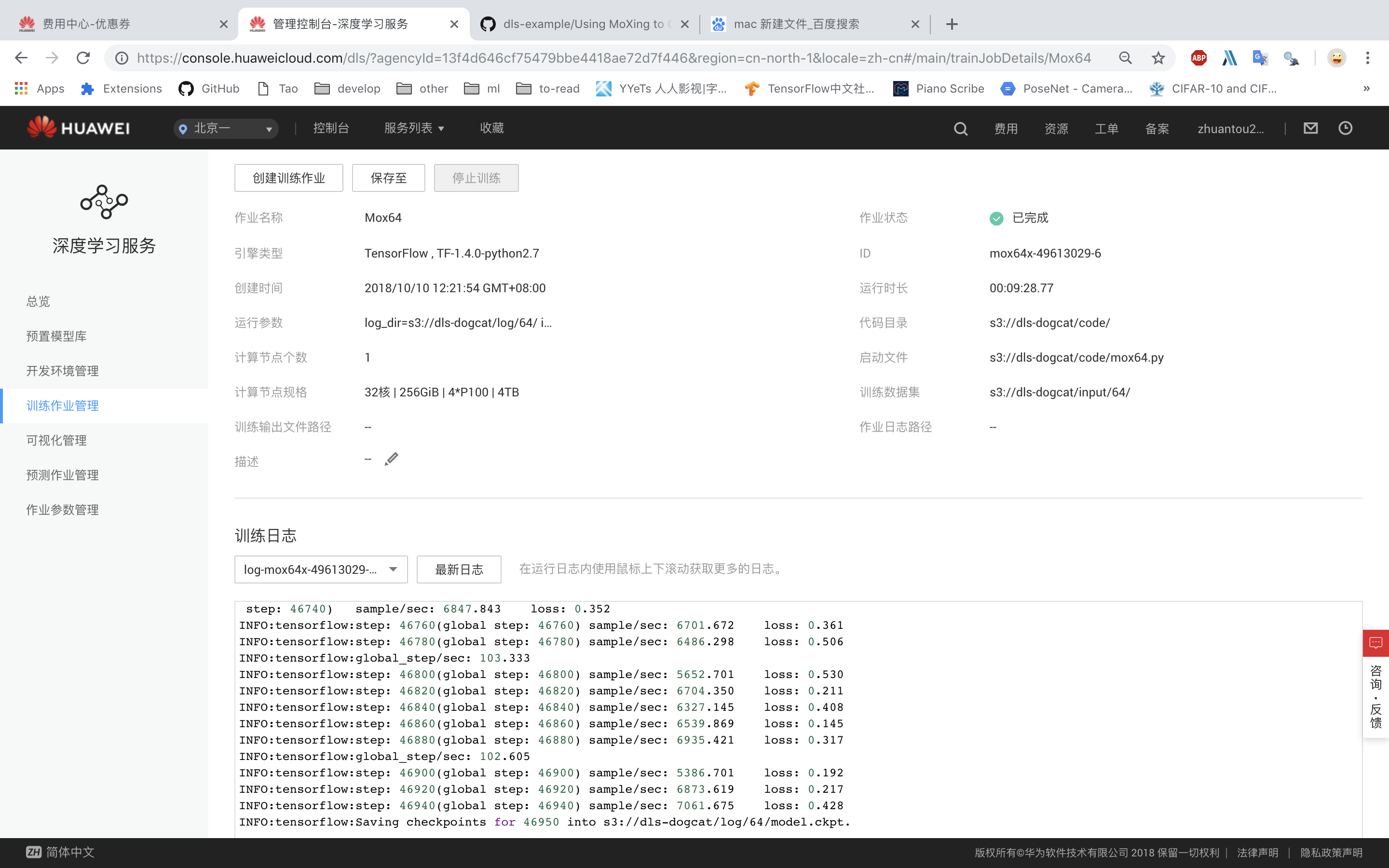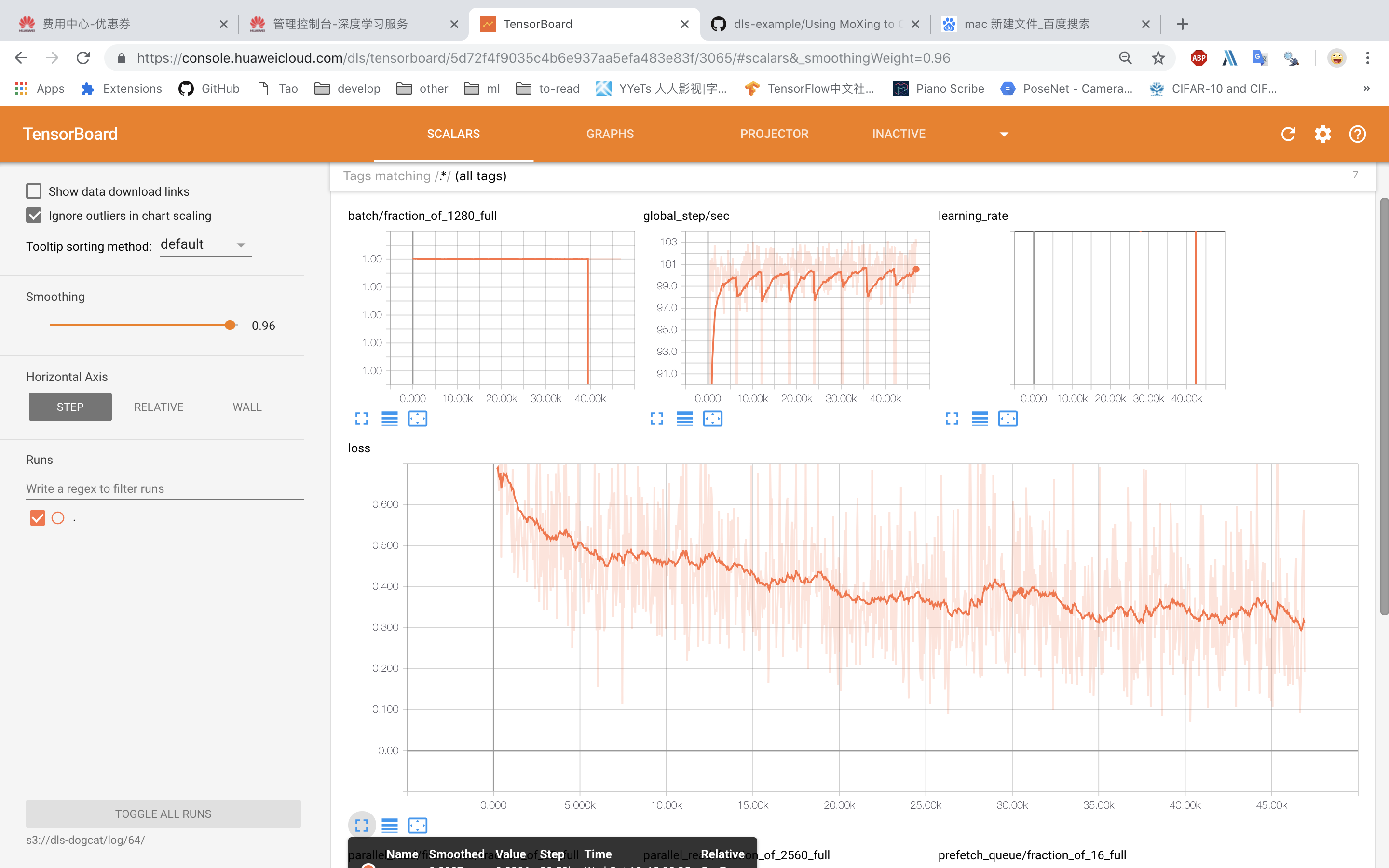Score: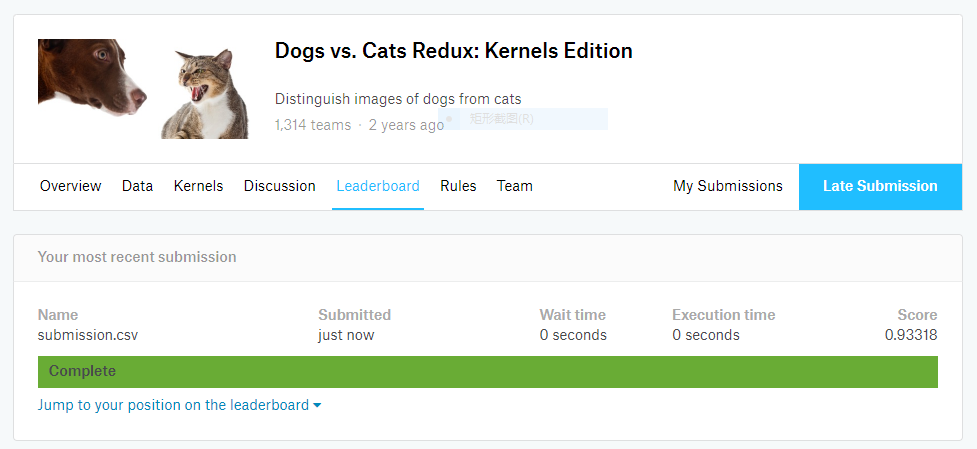Loss: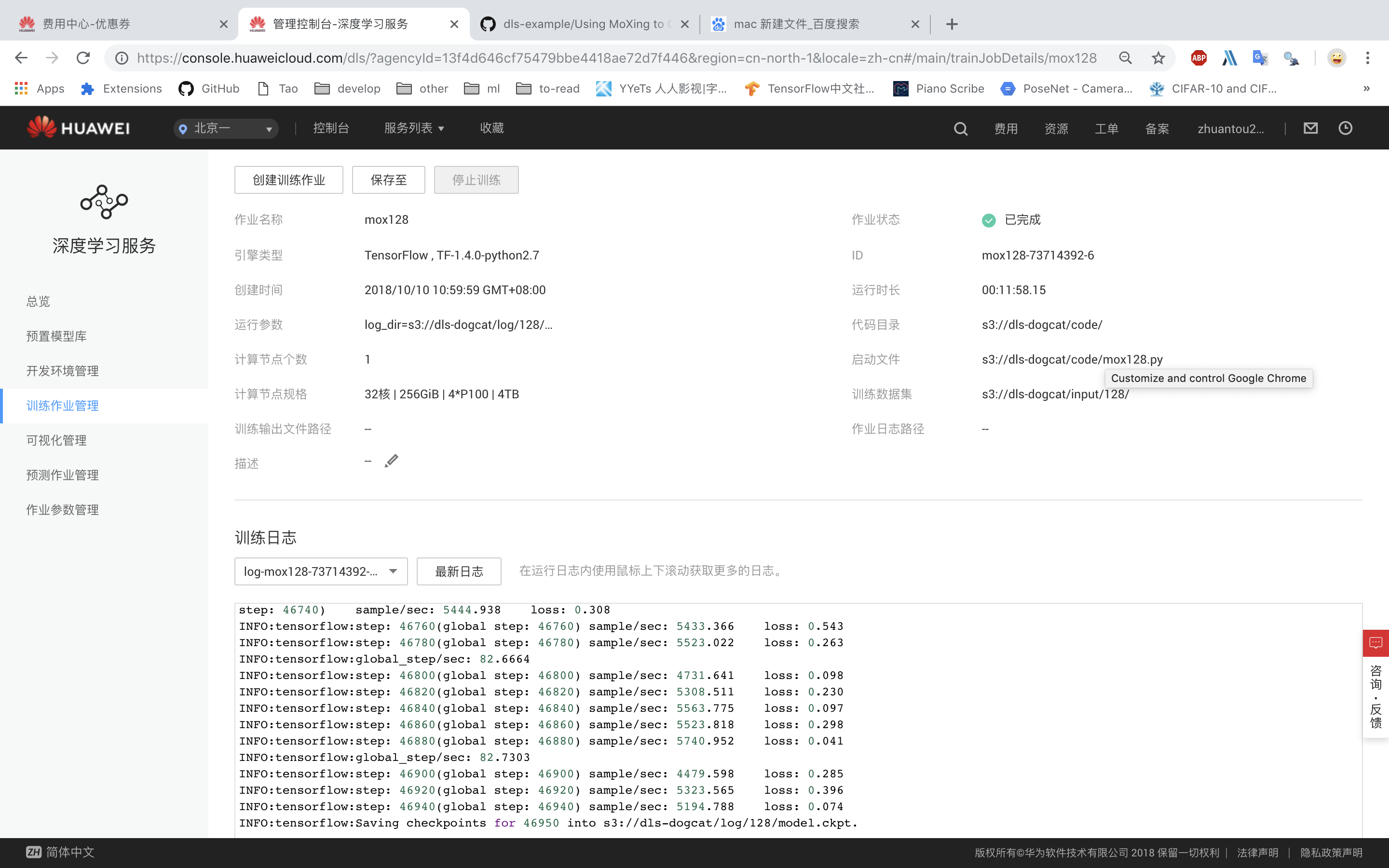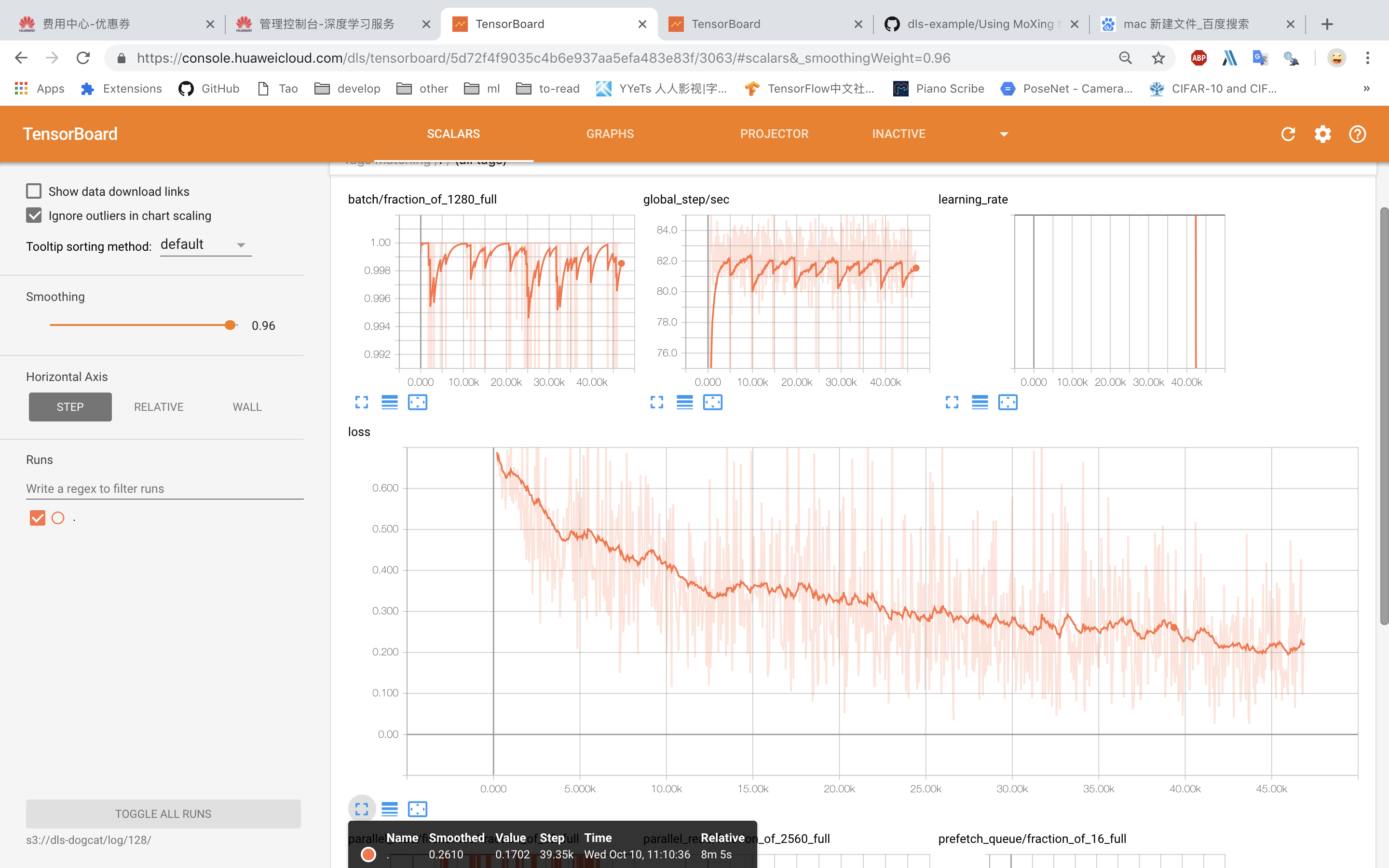Score: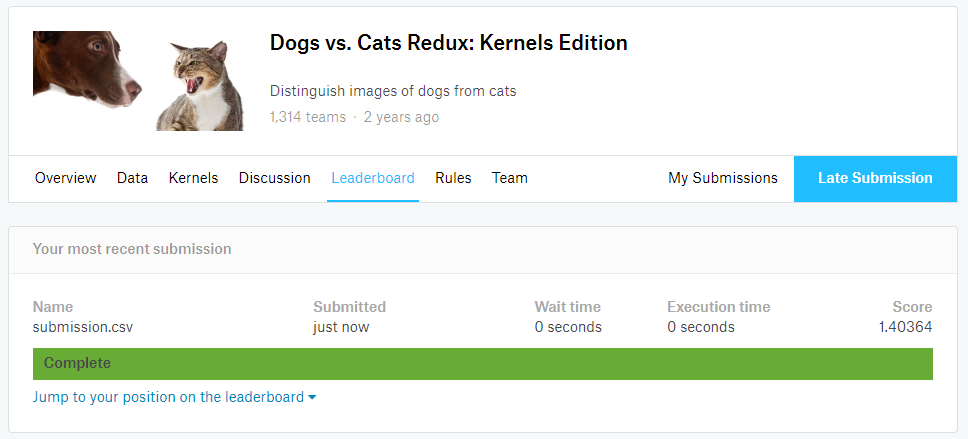1. 在CNN不变的情况下，128大小的训练可以达到更低的损失，但是似乎结果并不比64好，而且与keras对比，似乎这个训练过程中有过拟合的问题，减少max_number_of_steps应该能得到更好的效果；
2. 使用GPU的速度可快多了，最初的keras使用CPU跑了10小时以上，使用4个GPU可以在几十分钟内跑完更多轮的数据，效果相当的好。

## 总结

1. 进行图片识别时基本的方式是使用卷积神经网络，所以使用基于tensorflow的keras可以迅速搭建一个卷积神经网络。
2. 使用CNN的过程中可能会遇到内存不够的情况，一方面可以考虑优化CNN结构，另一方面可以考虑使用vgg16等已经训练好的模型加上自己的模型进行调试。
3. 使用华为云进行开发的过程中会遇到一些问题，比如读取图片的方式被限定，tensorflow1.8版本model使用dataset会报错，文件写入的方式被限定，notebook意外断开连接等等。
4. 使用华为云很方便，基于一个云平台基本可以完成所有的机器学习开发，而且提供了不少的学习工具，还是很实用的。
5. 希望华为云可以提供更加直接的云计算平台使用方式，就我所想的应该是提供一个可以ssh的服务器，这样代码迁移更加方便。

2019-05-19 00:05:15 jiaxianhua 阅读数 101
• ###### 深度学习框架Tensorflow案例实战视频课程

深度学习框架Tensorflow案例实战视频培训课程概况： Tensorflow是谷歌开源的深度学习（包括机器学习）框架，伴随着人工智能业的兴盛其大名早已响彻云霄。本课程从Tensorflow安装开始讲起，从基本计算结构到深度学习各大神经网络，全程案例代码实战，一步步带大家入门如何使用深度学习框架Tensorflow，玩转Tensorflow模型训练、等所有知识点。

30268 人正在学习 去看看 唐宇迪

• 你还可以了解在哪里可以 部署 你为数百万人服务的模型

• 查看 免费积分优惠 部分获得一些免费的 gpu 小时

# 云供应商 网站 价格 免费试用 / 免费积分
2 Kaggle Kernels https://www.kaggle.com 免费 永久免费*
4 FloydHub https://www.floydhub.com https://www.floydhub.com/pricing free 2 GPU powerups in 14 days trial plan
5 Onepanel https://www.onepanel.io https://www.onepanel.io/pricing -
6 Nimblebox https://nimblebox.ai https://nimblebox.ai/plans free \$5 worth of cloud credits
7 paperspace https://www.paperspace.com https://www.paperspace.com/pricing \$10 credits
8 Overture https://www.overture.ai - free credits on signup
9 Dataiku https://www.dataiku.com - Free Plans
10 Cloudalize https://www.cloudalize.com https://www.cloudalize.com/pricing/ -
11 Deepcognition https://deepcognition.ai https://deepcognition.ai/products Desktop version free to use
12 GPUeater https://gpueater.com https://gpueater.com/#pricing -
13 Vast.ai https://vast.ai https://vast.ai/console/create/ -
15 Snark https://snark.ai https://scale.snark.ai/pricing -
16 Crestle https://crestle.ai https://crestle.ai/#pricing 1 hour of free GPU usage on sign up fast.ai
17 Vector Dash(gaming) https://vectordash.com - free 7 day plan
18 Spell https://spell.run/developers https://spell.run/pricing \$10 GPU credit on signup
19 Rapid Switch https://www.rapidswitch.com pricing -
20 Salamander https://salamander.ai https://salamander.ai -
22 vscaler https://www.vscaler.com on request -
23 AWS Sagemaker https://aws.amazon.com/sagemaker/ pricing Free plans
24 Exoscale https://www.exoscale.com/gpu/ pricing -
25 Cirrascale http://www.cirrascale.com Work station -
26 Alibaba cloud https://alibabacloud.com pay as you go \$300 credits
27 IBM Cloud https://www.ibm.com/cloud/gpu pay as you go \$200 credits
29 Valohai https://valohai.com https://valohai.com/pricing/ free trial avaliable
30 Nvidia cloud https://www.nvidia.com/en-us/data-center/gpu-cloud-computing/ - -
31 One stop System https://www.onestopsystems.com - -
32 Azure https://azure.microsoft.com/en-in/services/machine-learning-studio/ pricing \$200 credits
33 Omnisci https://www.omnisci.com https://www.omnisci.com/cloud 14 day free trial
34 Rendsolve https://rendsolve.com https://rendsolve.com/pricing -
35 Golem https://golem.network - -

# 将模型部署为 Web 应用

# 供应商 网站 价格 免费试用 / 免费积分
1 Render https://render.com https://render.com/pricing -
2 Heroku https://www.heroku.com https://www.heroku.com/pricing Free plan (model<500MB)
3 Digtal Ocean https://www.digitalocean.com pay as you go free \$100 credits with github student pack
4 Glitch https://glitch.com - -
5 Zeit https://zeit.co https://zeit.co/pricing Free plan available

# 优惠

• Paperspace 提供 10 美元的免费 Gradient° 积分 fast.ai 链接

• 你是否有一个 GPU 躺在你的机器使用 Vast.ai 租来赚钱？

• 试驾 Nvidia GPU 链接

• Google Cloud Research 计划 - 提供 \$5000+ 积分 链接

• 用于研究的 AWS 云积分 - 链接

• Nvidia GPU Grant 计划 - 链接

• 如果您是初创公司，那么谷歌已经为您提供了启动计划，为您提供 1000-100000 美元的积分 - 链接

• Google 向研究人员提供 1000 TPU 的集群 总的来说，这个集群总共提供超过 180 petaflops 的初始计算能力 techcrunch 链接 - 应用程序链接

• Github 教育包 - 以及许多优惠，最高可获得 110 美元的 AWS 积分 - 链接

• 注意 fast.ai 论坛 获取免费积分的优惠券代码

• Valohai 为学生和研究人员提供 研究员许可证

• 想要使用 超级计算机 但还没有，去 Golem - Golem 是一个 分散的计算能力市场。它能够在 P2P 使用 CPU 和 GPU ，使应用程序所有者和个人用户能够从其他用户机器中租用资源，因此 turbo 会为您的下一次模型培训收费。

## 备注

• Google colab 和 Kaggle 内核的会话时间有限
• 大多数 gpu 提供程序运行在 AWS，GCP 等之上，因此可能与后者具有或多或少相同的定价
• 上面给出的信息最适合我的搜索能力，您可以与提供商重新检查定价和其他信息
• 许可证

2018-09-19 00:23:07 oliongs 阅读数 1235
• ###### 深度学习框架Tensorflow案例实战视频课程

深度学习框架Tensorflow案例实战视频培训课程概况： Tensorflow是谷歌开源的深度学习（包括机器学习）框架，伴随着人工智能业的兴盛其大名早已响彻云霄。本课程从Tensorflow安装开始讲起，从基本计算结构到深度学习各大神经网络，全程案例代码实战，一步步带大家入门如何使用深度学习框架Tensorflow，玩转Tensorflow模型训练、等所有知识点。

30268 人正在学习 去看看 唐宇迪

# 1. 整体框架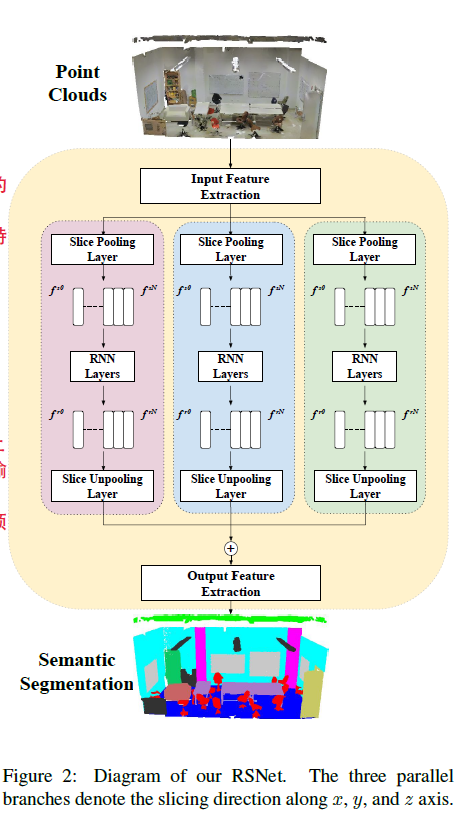# 2.局部依赖模块

## Slice Pooling Layer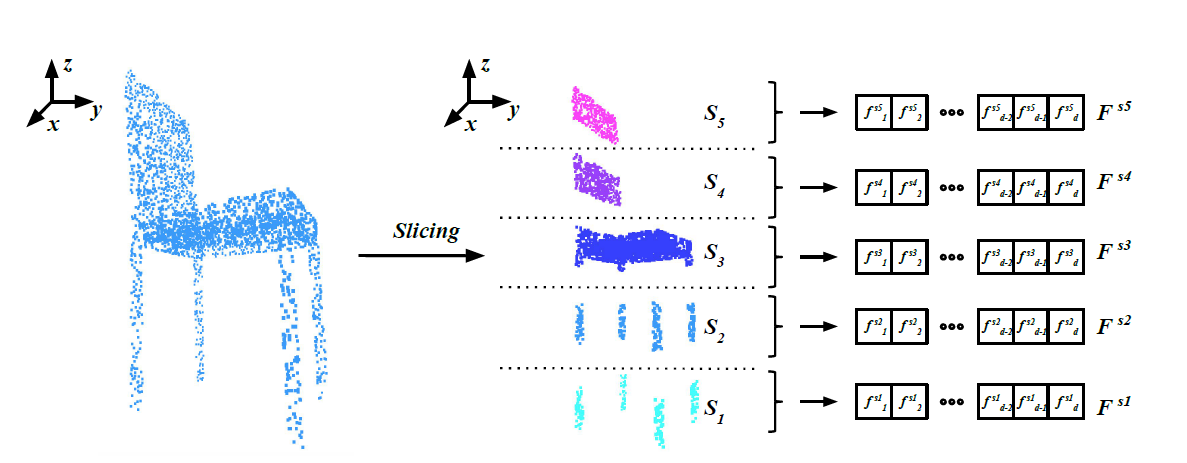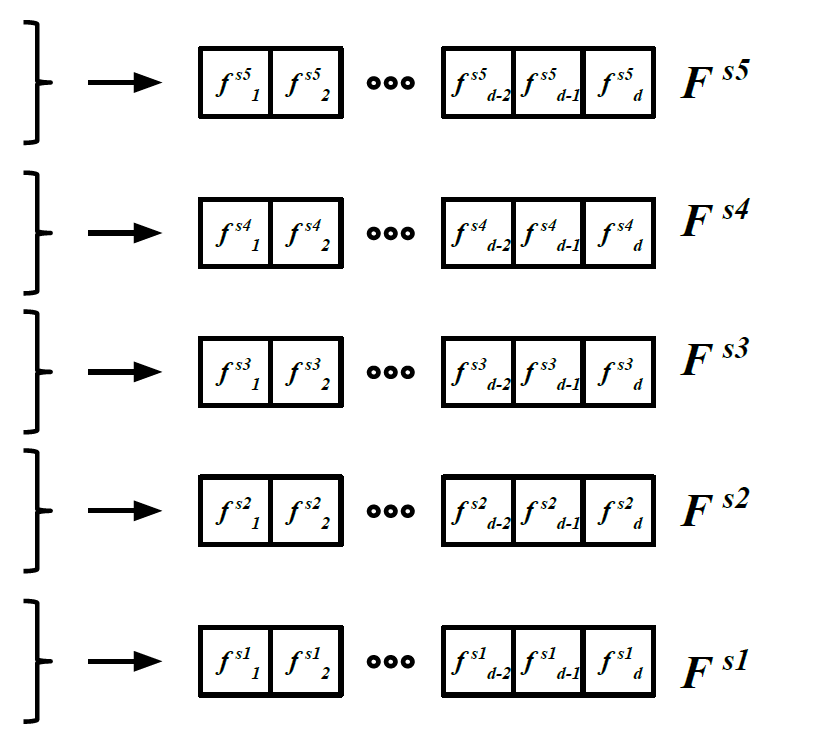## RNN Layer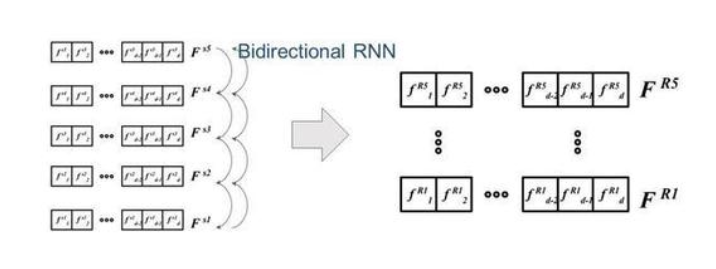## Slice Unpooling Layer